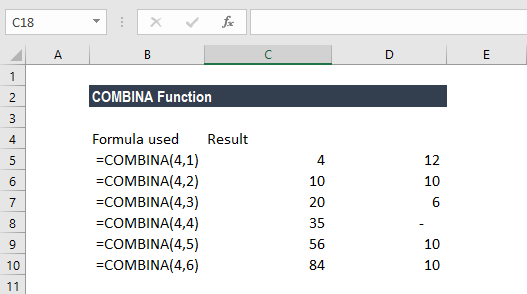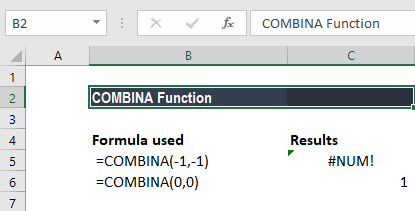# COMBINA Function

Calculate the number of combinations along with repetitions for a given number of items

## What is the COMBINA Function?

The COMBINA function is an Excel Math and Trigonometry function. It will calculate for a given number of items the number of combinations, along with repetitions. The function was introduced in MS Excel in 2013 and hence not available in earlier versions.

### Formula

=COMBINA(number, number_chosen)

The COMBINA function uses the following arguments:

1. Number (required argument) – This should be either greater than or equal to 0. Also, it should be greater than or equal to number_chosen. Non-integer values, if entered for this parameter, are truncated.
2. Number_chosen (required argument) – This must be greater than or equal to 0. Non-integer values are truncated.

### How to use COMBINA Function in Excel?

To understand the uses of the COMBINA function, let’s consider a few examples:

#### Example 1

Suppose we are given six numbers: 1, 2, 3, 4, 5, 6. Now let’s see how the COMBINA function calculates the number of combinations (with repetitions) for other numbers of objects taken from a set of 4.For any six objects – a, b, c, d, e, f – there are 21 different combinations (with repetitions) of 2 objects. These are:

aa, ab, ac, ad, ae, af, bb, bc, bd, be, bf, cc, cd, ce, cf, dd, de, df, ee, ef, ff

The COMBINA function helps derive such combinations. It uses the formula (n+m-1) ÷ (n-1) where n is the number and m is the number_chosen. Remember the results of COMBINA above include repetitions.

#### Example 2

Let’s see some interesting things about COMBINA. If you enter the following arguments in a COMBINA function, the results will be:COMBINA(0,0) is the only non-error output when n=0 yet this should be equal to COMBIN(-1,-1), which returns an error. It implies that the function includes an exception of n-m=0.

### Differences between COMBIN and COMBINA

The COMBIN and COMBINA functions differ in the following ways:

• COMBIN does not count repetitions whereas COMBINA does. For example, in a set of three objects (a, b, c), COMBINA will return the result 6; the combinations are aa, ab, ac, bb, bc, cc, whereas, COMBIN will return the result 3; the combinations are ab, ac, bc.
• The formulas used by the functions are different.

### Things to remember about the COMBINA Function

1. #VALUE! error – Occurs when either of the argument is non-numeric.
2. #NUM! error – Occurs when the value of either argument is outside of its constraint.

Thanks for reading CFI’s guide to important Excel functions! By taking the time to learn and master these functions, you’ll significantly speed up your financial analysis. To learn more, check out these additional CFI resources:

• Excel Functions for Finance